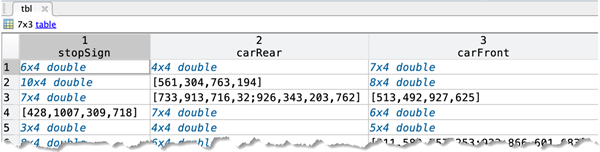evaluateDetectionMissRate

Evaluate miss rate metric for object detection

Description

example

logAverageMissRate = evaluateDetectionMissRate(detectionResults,groundTruthData) returns the log-average miss rate of the detectionResults compared to groundTruthData, which is used to measure the performance of the object detector. For a multiclass detector, the log-average miss rate is a vector of scores for each object class in the order specified by groundTruthData.

example

[logAverageMissRate,fppi,missRate] = evaluateDetectionMissRate(___) returns data points for plotting the log miss rate–false positives per image (FPPI) curve, using input arguments from the previous syntax.

[___] = evaluateDetectionMissRate(___,threshold) specifies the overlap threshold for assigning a detection to a ground truth box.

Examples

collapse all

This example shows how to evaluate miss rate of a pretrained YOLO v2 object detector.

Load the Vehicle Ground Truth Data

Load a table containing the vehicle training data. The first column contains the training images, the remaining columns contain the labeled bounding boxes.

trainingData = data.vehicleTrainingData(1:100,:);

Add fullpath to the local vehicle data folder.

Create an imageDatastore using the files from the table.

imds = imageDatastore(trainingData.imageFilename);

Create a boxLabelDatastore using the label columns from the table.

blds = boxLabelDatastore(trainingData(:,2:end));

Load the detector containing the layerGraph for trainining.

detector = vehicleDetector.detector;

Evaluate and Plot the Miss Rate Results

Run the detector with imageDatastore.

results = detect(detector, imds);

Evaluate the results against the ground truth data.

[am, fppi, missRate] = evaluateDetectionMissRate(results, blds);

Plot the log-miss-rate/FPPI curve.

figure;
loglog(fppi, missRate);
grid on
title(sprintf('Log Average Miss Rate = %.1f', am))stopSigns = stopSignsAndCars(:, 1:2);
stopSigns.imageFilename = fullfile(toolboxdir('vision'),'visiondata', ...
stopSigns.imageFilename);

Train an ACF based detector.

detector = trainACFObjectDetector(stopSigns,'NegativeSamplesFactor',2);
ACF Object Detector Training
The training will take 4 stages. The model size is 34x31.
Sample positive examples(~100% Completed)
Compute approximation coefficients...Completed.
Compute aggregated channel features...Completed.
--------------------------------------------
Stage 1:
Sample negative examples(~100% Completed)
Compute aggregated channel features...Completed.
Train classifier with 42 positive examples and 84 negative examples...Completed.
The trained classifier has 19 weak learners.
--------------------------------------------
Stage 2:
Sample negative examples(~100% Completed)
Found 84 new negative examples for training.
Compute aggregated channel features...Completed.
Train classifier with 42 positive examples and 84 negative examples...Completed.
The trained classifier has 20 weak learners.
--------------------------------------------
Stage 3:
Sample negative examples(~100% Completed)
Found 84 new negative examples for training.
Compute aggregated channel features...Completed.
Train classifier with 42 positive examples and 84 negative examples...Completed.
The trained classifier has 54 weak learners.
--------------------------------------------
Stage 4:
Sample negative examples(~100% Completed)
Found 84 new negative examples for training.
Compute aggregated channel features...Completed.
Train classifier with 42 positive examples and 84 negative examples...Completed.
The trained classifier has 61 weak learners.
--------------------------------------------
ACF object detector training is completed. Elapsed time is 24.9453 seconds.

Create a struct array to store the results.

numImages = height(stopSigns);
results(numImages) = struct('Boxes', [], 'Scores', []);

Run the detector on the training images.

for i = 1 : numImages
[bboxes, scores] = detect(detector, I);
results(i).Boxes = bboxes;
results(i).Scores = scores;
end

results = struct2table(results);

Evaluate the results against the ground truth data.

[am, fppi, missRate] = evaluateDetectionMissRate(results, stopSigns(:, 2));

Plot log-miss-rate/FPPI curve.

figure
loglog(fppi, missRate);
grid on
title(sprintf('log Average Miss Rate = %.1f', am))Input Arguments

collapse all

Object locations and scores, specified as a two-column table containing the bounding boxes and scores for each detected object. For multiclass detection, a third column contains the predicted label for each detection. The bounding boxes must be stored in an M-by-4 cell array. The scores must be stored in an M-by-1 cell array, and the labels must be stored as a categorical vector.

When detecting objects, you can create the detection results table by using imageDatastore.

ds = imageDatastore(stopSigns.imageFilename);
detectionResults = detect(detector,ds);

Data Types: table

Labeled ground truth, specified as a datastore or a table.

Each bounding box must be in the format [x y width height].

• Datastore — A datastore whose read and readall functions return a cell array or a table with at least two columns of bounding box and labels cell vectors. The bounding boxes must be in a cell array of M-by-4 matrices in the format [x,y,width,height]. The datastore's read and readall functions must return one of the formats:

• {boxes,labels} — The boxLabelDatastore creates this type of datastore.

• {images,boxes,labels} — A combined datastore. For example, using combine(imds,blds).

• Table — One or more columns. All columns contain bounding boxes. Each column must be a cell vector that contains M-by-4 matrices that represent a single object class, such as stopSign, carRear, or carFront . The columns contain 4-element double arrays of M bounding boxes in the format [x,y,width,height]. The format specifies the upper-left corner location and size of the bounding box in the corresponding image.Overlap threshold for a detection assigned to a ground truth box, specified as a numeric scalar. The overlap ratio is computed as the intersection over union.

Output Arguments

collapse all

Log-average miss rate metric, returned as either a numeric scalar or vector. For a multiclass detector, the log-average miss rate is returned as a vector of values that correspond to the data points for each class.

False positives per image, returned as either a vector of numeric scalars or as a cell array. For a multiclass detector, the FPPI and log miss rate are cell arrays, where each cell contains the data points for each object class.

Log miss rate, returned as either a vector of numeric scalars or as a cell array. For a multiclass detector, the FPPI and log miss rate are cell arrays, where each cell contains the data points for each object class.